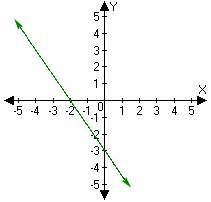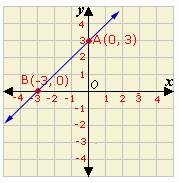Definition OfIntercept

The point at which a curve intersects an axis is known as an Intercept

The x-intercept is the point where the curve crosses the x-axis and the y-intercept is the point where the curve crosses the y-axis.

Example of InterceptIn the figure, the line cuts the x-axis at (- 2, 0) and y-axis at (0, - 3). So, x-intercept is - 2 and y-intercept is - 3.

Solved Example on Intercept

Ques: Find the x and y intercepts of the line AB shown below.Choices:

A. x-intercept is - 3 and y-intercept is 0
B. x-intercept is 3 and y-intercept is - 3
C. x-intercept is - 3 and y-intercept is 3
D. x-intercept is 3 and y-intercept is 3